## Why hillwalkers should love the Comte de Buffon

Wandering the mountains of the UK has been a big part of my life. You won’t be surprised that before I start a long walk I like to know roughly what I’m letting myself in for. One part of this is estimating how far I’ll be walking.

Several decades ago my fellow student and hillwalking friend David told me of a quick and simple way to estimate the length of a walk. It uses the grid of kilometre squares that is printed on the the Ordnance Survey maps that UK hillwalkers use.

To estimate the length of the walk, count the number of grid lines that the route crosses, and divide by two. This gives you an estimate of the length of the walk in miles.

Yes, miles, even though the grid lines are spaced at kilometre intervals. On the right you can see a made-up example. The route crosses 22 grid lines, so we estimate its length as 11 miles.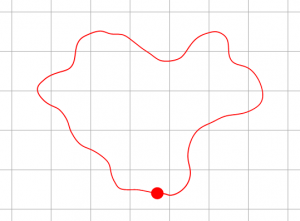Is this rule practically useful? Clearly, the longer a walk, the more grid lines the route is likely to cross, so counting grid lines will definitely give us some kind of measure of how long the walk is. But how good is this measure, and why do we get an estimate in miles when the grid lines are a kilometre apart?

I’ve investigated the maths involved, and here are the headlines. They are valid for walks of typical wiggliness; the rule isn’t reliable for a walk that unswervingly follows a single compass bearing.

• On average, the estimated distance is close to the actual distance: in the long run the rule overestimates the lengths of walks by 2.4%.
• There is, of course, some random variation from walk to walk. For walks of about 10 miles, about two-thirds of the time the estimated length of the walk will be within 7% of the actual distance.
• The rule works because 1 mile just happens to be very close to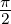kilometres.

The long-run overestimation of 2.4% is tiny: it amounts to only a quarter-mile in a 10-mile walk. The variability is more serious: about a third of the time the estimate will be more than 7% out. But other imponderables (such as the nature of the ground, or getting lost) will have a bigger effect than this on the effort or time needed to complete a walk, so I don’t think it’s a big deal.

In conclusion, for a rule that is so quick and easy to use, this rule is good enough for me. Which is just as well, because I’ve been using it unquestioningly for the past 35 years.

#### And the Comte de Buffon?

George-Louis Leclerc, Comte de Buffon,  (1707-1788) was a French intellectual with no recorded interest in hillwalking. But he did consider the following problem, known as Buffon’s Needle:

Suppose we have a floor made of parallel strips of wood, each the same width, and we drop a needle onto the floor. What is the probability that the needle will lie across a line between two strips?

Let’s recast that question a little and ask: if the lines are spaced 1 unit apart, and we drop the needle many times, what’s the average number of lines crossed by the dropped needle? It turns out that it iswhere l is the length of the needle. Now add another set of lines at right angles (as if the floor were made of square blocks rather than strips). The average number of lines crossed by the dropped needle doubles toCan you see the connection with the distance-estimating rule? The cracks in the floor become the grid lines, and the needle becomes a segment of the walk. A straight segment of a walk will cross, on average,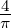grid lines per kilometre of its length. Now a mile is 1.609 kilometres, so a segment of the walk will, on average, cross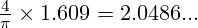grid lines per mile, which is very close to 2 grid lines per mile, as our rule assumes. If a mile werekm (1.570… km), we’d average exactly 2 grid lines per mile.

So the fact that using a kilometre grid gives us a close measure of distance in miles is just good luck. It’s because a mile is very close tokilometres.

In the next post, I’ll explore the maths further. We’ll see where the results above come from, and look in more detail at walk-to-walk variability. We’ll also see why results that apply to straight lines also apply to curved lines (like walks), and in doing so discover that not only did the Comte de Buffon have a needle, he also had a noodle.

Mathematical typesetting by QuickLaTeX.

## Ink cubedEdwin Pickstone, a colleague at Glasgow School of Art, appointed me as a maths consultant recently. His project was to produce a book which had a black square on each page. The size of the square and the number of pages had to be such that the envelope of the resulting block of ink was a cube containing 1 kilogram of ink.

His printer provided a sample run so that we knew what the areal density of the ink film would be, and I did the sums to work out how big the squares would need to be and how many pages we’d need. It turned out that we needed squares of side 19.28 cm, and (coincidentally) 1928 pages.

The responsibility weighed heavily on me, and I was a little nervous as the order went off to the printer. So I was relieved and delighted when Edwin told me that when he’d walked into the printers to pick the job up, the printer said ‘bloody hell, it took a whole kilo tin of ink to print your job’.

Edwin is Lecturer, Typography Technician and Designer in Residence at Glasgow School of Art.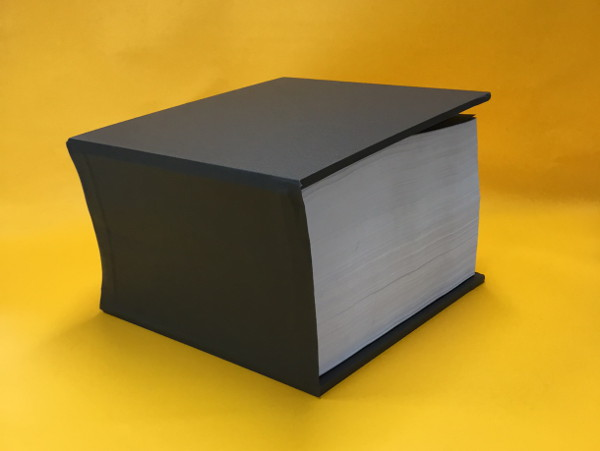## Walking bass

How fast does a plucked guitar string move? It’s a complete blur, so surely it’s travelling at a terrific speed. 50 miles per hour? 100 miles per hour? What do you think?

Walking pace. A typical speed for the middle of a guitar string given a good twang is walking pace. And that’s the middle of the string. Near its ends, it’ll be moving much more slowly.

How can that be so? Well, although the string is going back and forth hundreds of times a second, it’s only travelling a few millimetres on each trip. So the distance that it travels in each second isn’t as much as you might expect. It certainly isn’t as far as I expected.

If you think that the string moves slowly, what about the body of the guitar? The string itself radiates very little sound into the air; its job is to set the body of the guitar vibrating. The body of the guitar, with its much larger area, is much more effective than the string at setting air into motion. Yet we can’t even see the body vibrating. At what snail’s pace must it be moving?

Remember also that the air molecules on which the guitar body acts are already travelling at something like 500 metres per second. Isn’t it astonishing that the sub-pedestrian movements of the guitar affect the movement of the air molecules enough to produce a sound that we can easily hear?

#### The calculation

Suppose that we look near the centre of the string, where its movement is the greatest. Shortly after being plucked, the width of the blur that we see is going to be something like 5 mm. So for every complete oscillation, the string does a round trip of about 10 mm.

The frequencies of the strings on a standard 6-string acoustic guitar are (to the nearest whole number) 82, 110, 147, 196, 247 and 330 hertz (one hertz is one oscillation per second). If we multiply these frequencies by the 10 mm round trip, it tells us how far the centre of each string travels in one second, that is, its average speed. I’ve converted these speeds into metres per second. For comparison, a brisk walk at 4 mph is about 1.8 ms-1.

Note nameMean speed of middle of string in metres per second (to 2 sig. fig.)
E0.82
A1.1
D1.5
G2.0
B2.5
E3.3

Our brisk walk is right in the middle of this range. And remember, we’ve done the calculation for the part of each string that’s moving the most. Near its ends, each string will be moving much more slowly than this.

#### Complication 1 – how long is the round trip really?

So far, we’ve assumed that each part of the string does a simple back-and-forth movement along a straight line, but if you carefully watch a vibrating guitar string you’ll see that the string often moves in an irregular but roughly elliptical orbit. The wire-wound lower strings show this most clearly; you can see a hint of it in the image at the top. This makes the round-trip distance a bit longer than the 10 mm that we used in the calculation earlier. Does this affect the string’s average speed much?

We’ll take the extreme case where each part of the string moves at constant speed in a circle of diameter 5 mm rather than along a straight line 5 mm long. The circumference of this circle will bemm, or about 15 mm. So the speeds of the strings (in this rather unlikely extreme case) will be about 50% greater than the ones listed above. They are still hardly impressive.

#### Complication 2 – the peak speed

So far, we’ve calculated the mean (average) speed of the string over its round trip. However, unless it’s moving in a perfect circle, its speed changes constantly, and its peak speed will be higher than its mean speed. How much higher?

Imagine that part of the string is vibrating back and forth along a straight line in the simplest possible way. At one end of the movement the string is stationary as it changes direction. It then speeds up, reaching its peak speed at the centre of its range of movement. Then it slows down until it reaches a halt again at the other end of the movement and changes direction again. How do we calculate the peak speed if we know the time taken for the round trip?

You’ll need to know a bit of maths for the next bit. The simplest vibration of the string is where each part undergoes simple harmonic motion, that is, where its position varies sinusoidally with time. This means in turn that the velocity of the string also varies sinusoidally in time. So we need to ask: how does the mean value of a sinusoid compare to its peak value?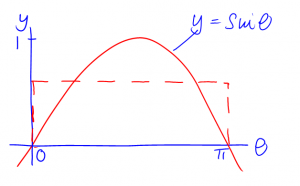Consider the function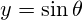, for half a cycle, that is, for theta from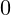to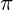radians. We construct a rectangle between these limits, such the rectangle’s area equals the area under the sine curve. The mean value of the sine function is the height of the rectangle, which its area divided by its width (which is). So we need to work out the areaunder the sine curve betweenand, which we can do by integration: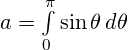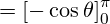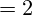We constructed the rectangle to have area. Its width is, so its height, and therefore the mean value of the sine function, is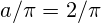. The height to the peak of the sine curve is 1, so the peak value of the sine function is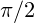times its mean value. This means that the peak speed of our guitar string istimes, or 50% more than, its average speed. Again, nothing to write home about.

(Rather pleasingly, this ratio ofis the same ratio that we got earlier in Complication 1. It means that the peak speed of a particle doing simple harmonic motion with a given amplitudeand period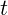is exactly the same as the (constant) speed of a particle moving in a circular orbit of radiuswith period. This isn’t a coincidence. It arises because the circular motion can be considered as two linear simple harmonic motions at right angles to each other.)